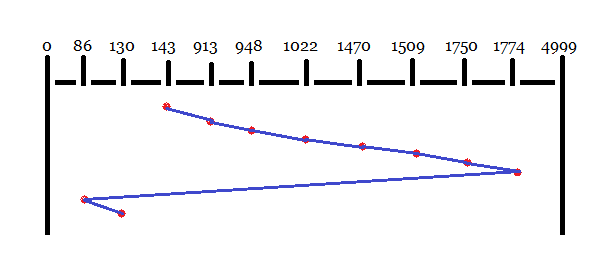### Disk scheduling numericals

Prob. A 99 disk drive has 100 cylinders, numbered 0 to 99. Disk requests come to the disk drives for cylinders 12, 26, 24, 4, 42, 8 and 50 in that order. The driver is currently serving a request at cylinder 24. A seek takes 6 msec per cylinder moved. How much seek time is needed for the shortest seek time first (SSTF) algorithm?

Sol. SSTF: The movement of disk head from 143 to others is as shown in diagram.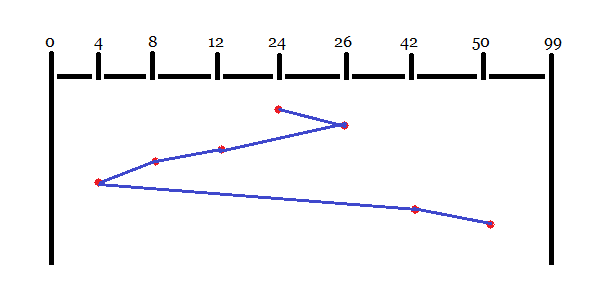So, total head movement = 02+14+04+04+38+08=70 cylinders.
Seek time = 70 X 6 = 420 msec = 0.42 sec

Prob. Suppose that a disk drive has 5000 cylinders numbered 0 to 4999. The drive is currently serving a request at cylinder 143. The queue of pending requests in FIFO order is- 86, 1470, 913, 1774, 948, 1509, 1022, 1750, 130. Starting from the current head position, what is the total distance that the disk arm moves to satisfy all the pending requests for each of the following disk scheduling algorithms?
(i)                FCFS
(ii)              SSTF
(iii)            SCAN
(iv)            C-SCAN
(v)              C-LOOK
Sol.
(i). FCFS: The movement of disk head from 143 to others is as shown in diagram.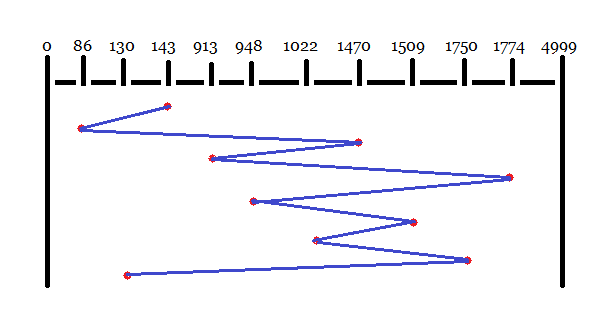So, total head movement = 57+1384+557+861+826+561+487+728+1620=7081 cylinders.
(ii). SSTF: The movement of disk head from 143 to others is as shown in diagram.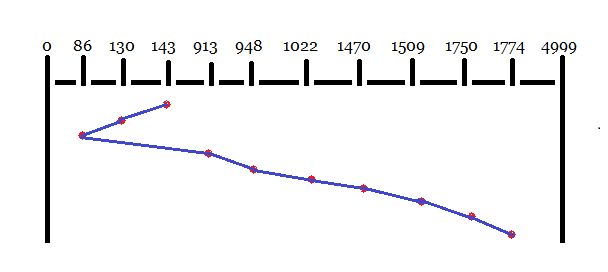So, total head movement = 13+44+827+35+74+448+39+241+24=1745 cylinders.
(iii). SCAN: The movement of disk head from 143 to others is as shown in diagram.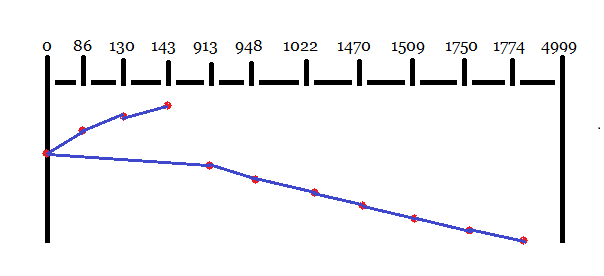So, total head movement = 13+44+86+913+35+74+448+39+241+24=1917 cylinders.
(iv). C-SCAN: The movement of disk head from 143 to others is as shown in diagram.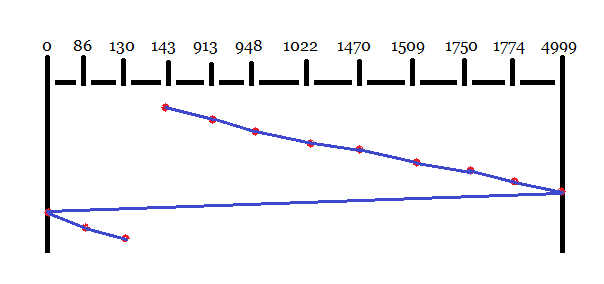(V). C-LOOK/LOOK: The movement of disk head from 143 to others is as shown in diagram.